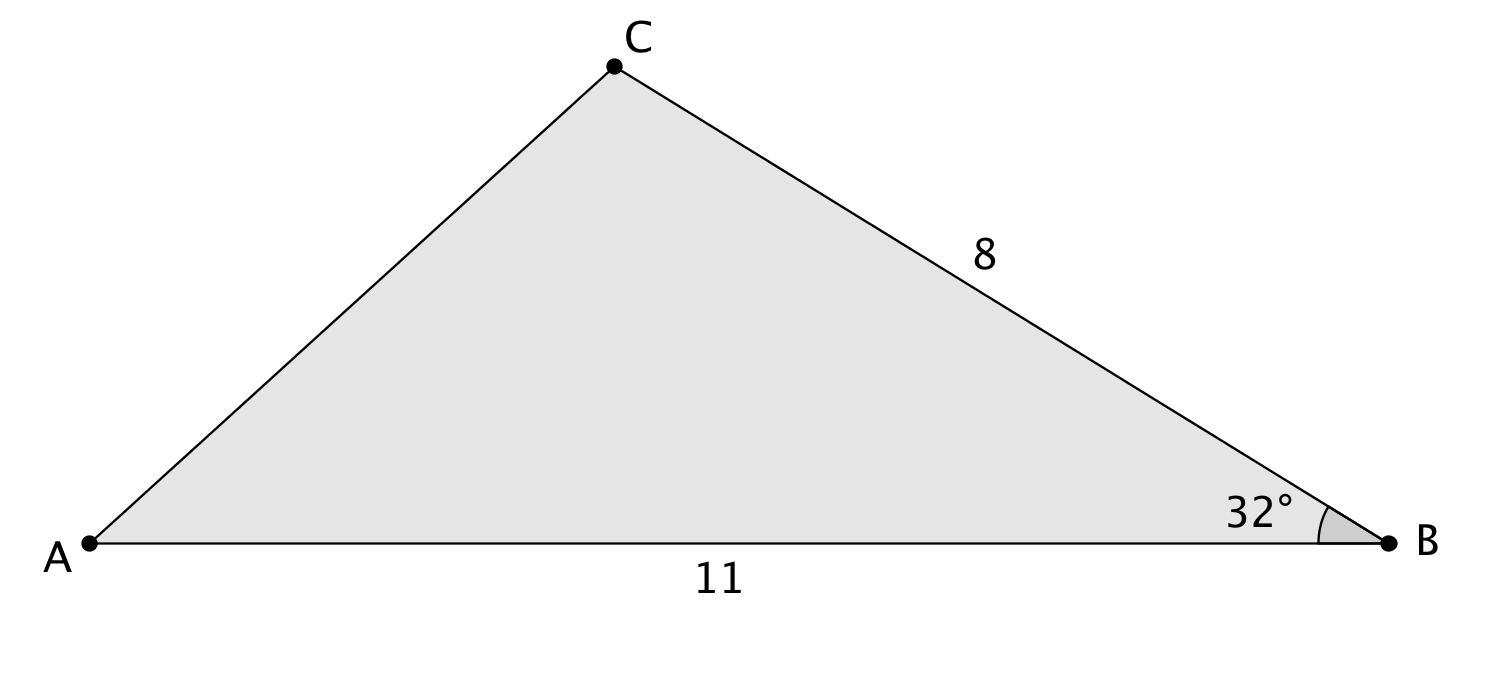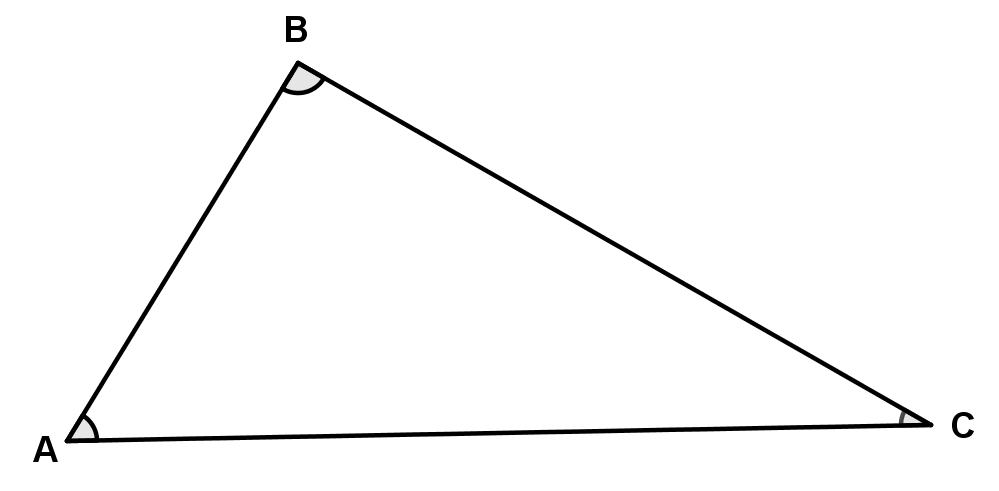# Right Triangles and Trigonometry

Lesson 16

Math

Unit 4

Lesson 16 of 19

## Objective

Derive the area formula for any triangle in terms of sine.

## Common Core Standards

### Core Standards

• G.SRT.D.9 — Derive the formula A = 1/2 ab sin(C) for the area of a triangle by drawing an auxiliary line from a vertex perpendicular to the opposite side.

• 6.G.A.1

## Criteria for Success

1. Use the area formula for triangles to determine missing information in non-right triangles.
2. Identify that an auxiliary altitude is necessary in non-right triangles to represent the height.
3. Write the measurement of the height of a non-right triangle in terms of sine of the angle opposite to the height.
4. Assign the variables $A$, $B$, and $C$ to each of the vertices of a non-right triangle.
5. Assign the variables $a$$b$, and $c$ to each side opposite vertices $A$, $B$, and $C$ respectively in non-right triangles.
6. Generalize the area formula for non-right triangles as $\mathrm{Area}=\frac{1}{2}ab\mathrm{sin}(C)$.
7. Verify that the formula works for any non-right triangle once $a$$b$, and $c$  sides and $A$, $B$, and $C$ angles are defined.

## Tips for Teachers

This post on Math Central is helpful in developing knowledge on the area formula in terms of sine for triangles.

Fishtank Plus

Unlock features to optimize your prep time, plan engaging lessons, and monitor student progress.

## Anchor Problems

### Problem 1

Find the area of the following triangle.### Problem 2

Write a formula for the area of this non-right triangle.## Problem Set

The following resources include problems and activities aligned to the objective of the lesson that can be used to create your own problem set.

• Include problems where the students are given the area and need to find missing information about the non-right triangle.
• Include problems where the students are given the area and need to find missing information about the non-right triangle.

Explain why ${\frac{1}{2}ab\mathrm{sin}(C)}$ gives the area of a triangle with sides $a$ and $b$ and included angle $C$.

#### References

EngageNY Mathematics Precalculus and Advanced Topics > Module 4 > Topic B > Lesson 7Exit Ticket, Question #2

Precalculus and Advanced Topics > Module 4 > Topic B > Lesson 7 of the New York State Common Core Mathematics Curriculum from EngageNY and Great Minds. © 2015 Great Minds. Licensed by EngageNY of the New York State Education Department under the CC BY-NC-SA 3.0 US license. Accessed Dec. 2, 2016, 5:15 p.m..

Modified by Fishtank Learning, Inc.

Lesson 15

Lesson 17

## Lesson Map

Topic A: Right Triangle Properties and Side-Length Relationships

Topic B: Right Triangle Trigonometry

Topic C: Applications of Right Triangle Trigonometry

Topic D: The Unit Circle

Topic E: Trigonometric Ratios in Non-Right Triangles